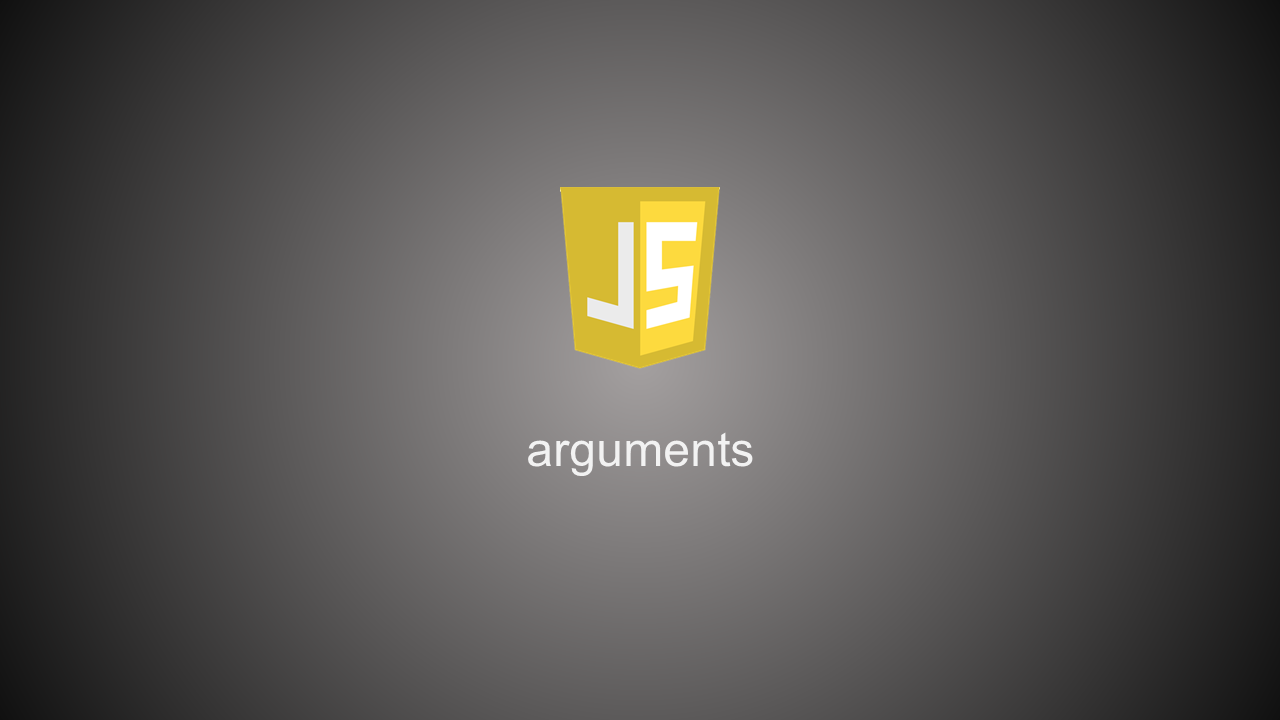## 1. 什么是 arguments

MDN 上解释：

arguments 是一个类数组对象。代表传给一个function的参数列表。

``````function printArgs() {
console.log(arguments);
}
printArgs("A", "a", 0, { foo: "Hello, arguments" });
``````

``["A", "a", 0, Object]``

## 2. arguments 操作

2.1 arguments length

arguments 是个类数组对象，其包含一个 `length` 属性，可以用 `arguments.length` 来获得传入函数的参数个数。

``````function func() {
console.log("The number of parameters is " + arguments.length);
}

func();
func(1, 2);
func(1, 2, 3);
``````

``````The number of parameters is 0
The number of parameters is 2
The number of parameters is 3
``````

2.2 arguments 转数组

``Array.prototype.slice.call(arguments);``

``[].slice.call(arguments);``

``````const obj = { 0: "A", 1: "B", length: 2 };
const result = [].slice.call(obj);
console.log(Array.isArray(result), result);
``````

``````true ["A", "B"]
``````

``````// Leaking arguments example1:
function getArgs() {
return arguments;
}

// Leaking arguments example2:
function getArgs() {
const args = [].slice.call(arguments);
return args;
}

// Leaking arguments example3:
function getArgs() {
const args = arguments;
return function() {
return args;
};
}
``````

``````function getArgs() {
const args = new Array(arguments.length);
for(let i = 0; i < args.length; ++i) {
args[i] = arguments[i];
}
return args;
}
``````

2.3 修改 arguments 值

``````function foo(a) {
"use strict";
console.log(a, arguments);
a = 10;
console.log(a, arguments);
arguments = 20;
console.log(a, arguments);
}
foo(1);
``````

``````1 1
10 1
10 20
``````

``````function foo(a) {
console.log(a, arguments);
a = 10;
console.log(a, arguments);
arguments = 20;
console.log(a, arguments);
}
foo(1);
``````

``````1 1
10 10
20 20
``````

2.4 将参数从一个函数传递到另一个函数

``````function foo() {
bar.apply(this, arguments);
}
function bar(a, b, c) {
// logic
}
``````

2.5 arguments 与重载

``````function add(num1, num2) {
console.log("Method one");
return num1 + num2;
}

console.log("Method two");
return num1 + num2 + num3;
}

``````

``````Method two
Method two
``````

``````function add(num1, num2, num3) {
if (arguments.length === 2) {
console.log("Result is " + (num1 + num2));
}
else if (arguments.length === 3) {
console.log("Result is " + (num1 + num2 + num3));
}
}

``````

``````Result is 3
Result is 6
``````

## 3. ES6 中的 arguments

3.1 扩展操作符

``````function func() {
console.log(...arguments);
}

func(1, 2, 3);
``````

``1 2 3``

3.2 Rest 参数

``````function func(firstArg, ...restArgs) {
console.log(Array.isArray(restArgs));
console.log(firstArg, restArgs);
}

func(1, 2, 3);
``````

``````true
1 [2, 3]
``````

3.3 默认参数

``````function func(firstArg = 0, secondArg = 1) {
console.log(arguments, arguments);
console.log(firstArg, secondArg);
}

func(99);
``````

``````99 undefined
99 1
``````

3.4 arguments 转数组

`Array.from()` 是个非常推荐的方法，其可以将所有类数组对象转换成数组。

## 4. 数组与类数组对象

``````const obj = { 0: "a", 1: "b" };
const arr = [ "a", "b" ];
``````

``````function Foo() {}
Foo.prototype = Object.create(Array.prototype);

const foo = new Foo();
foo.push('A');
console.log(foo, foo.length);
console.log("foo is an array? " + Array.isArray(foo));
``````

``````["A"] 1
foo is an array? false
``````

``````function Bar() {}
Bar.prototype.push = Array.prototype.push;

const bar = new Bar();
bar.push('A');
bar.push('B');
console.log(bar);
``````

``````Bar {0: "A", 1: "B", length: 2}
``````